# Sum of Years Depreciation (SYD)

An accelerated depreciation for calculating an asset

The sum of years digit (SYD) is an accelerated depreciation for calculating an asset. This method takes the asset's useful life and adds it together to give the final depreciation expense amount.If the asset's useful life is five years, the sum of the years digit would be 5 + 4 + 3 + 2 + 1 = 15.

It is similar to the double-declining balancing method (DDB). However, its main aim is to depreciate an asset at an accelerated level. Companies choose this method because it provides a bigger depreciation tax shield in the first few years of an asset's life.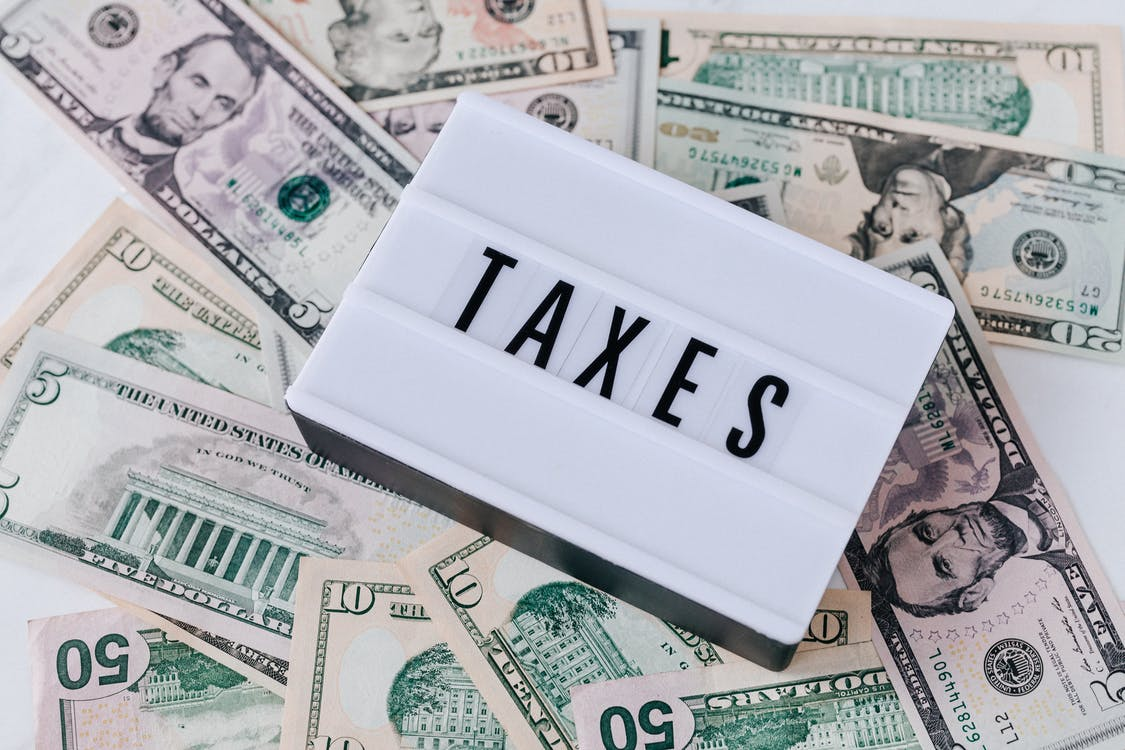To generate higher tax savings and benefit from the consequent overstated net income figure presented on the company's financial statements in the first few years of holding the assets, organizations in harsh tax regimes may choose to depreciate their assets more quickly.

The net income will be overestimated in later years when the depreciation amount is reduced.The sum of years method matches the cost of an asset and the overall use of an asset across the useful life of an asset. The advantage of this method is that the asset performance will be more productive in the early years and reduce over the years.

Therefore, it can charge higher depreciation in the early years and decrease in the later years.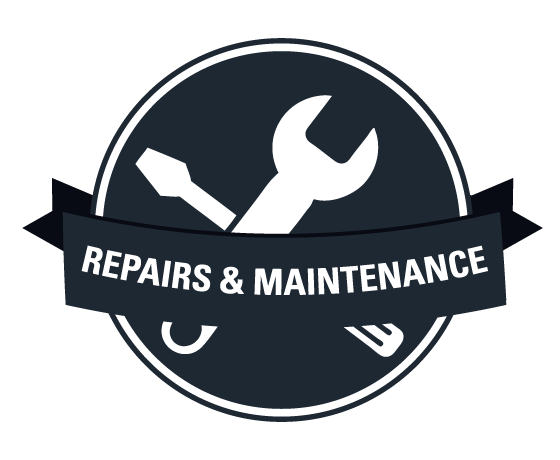When an asset gets deteriorates, it requires higher repairs and maintenance charges. So to balance the cost of the maintenance of an asset, it decreases its depreciation charges. Therefore, the earnings may be distorted if the year's sum is not a method of accelerated depreciation.

In years to come, the depreciation expense may not counterbalance the cost of repairs and maintenance.

For example, if an asset's useful life is five years, the sum of the years digit would be 5 + 4 + 3 + 2 + 1 = 15. Every digit is divided by its total to get the depreciation percentage each year.

The depreciation percentage for each year is calculated by the years remaining in the useful life divided by the sum of the remaining life every year throughout the asset's life.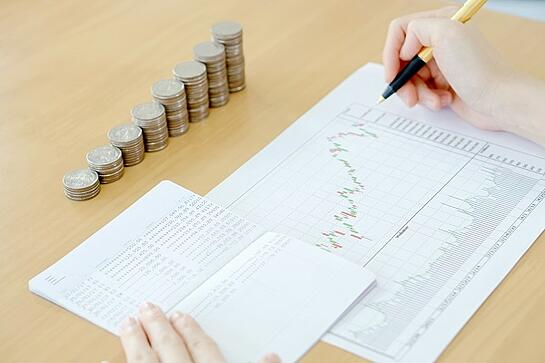In every method of depreciation, it would result in almost the same answer, but the difference is that of time taken for depreciation.

To calculate depreciation, the straight-line method would take much longer, the double declining balancing method is similar to the sum of years digit, and the production unit requires the number of units. This method facilitates the calculation when the performance of an asset is at its highest.

This method includes electronics such as computers, mobile phones, and the automobile industry. However, when an advent of new technology or a new model of a car like Tesla comes in place, all these examples mentioned above can quickly be outdated.

## The formula for Sum Of The Years Digits Depreciation

The sum of years digit assumes that the asset is at its highest in the initial years, is more productive, and decreases in subsequent years.

Depreciation = (Remaining Use life of an Asset/ Sum of Years Digit) * Depreciable Amount

The steps used are:

1. Determine the depreciable amount

2. Calculate the sum of the useful life of an asset

3. Multiply depreciable amount with depreciation

4. Calculate depreciation for each year

Let's take an example of a worldwide known coffee company and explain it step by step### Everything You Need To Build Your Accounting Skills

To Help You Thrive in the Most Flexible Job in the World.

Step 1: Determine the depreciable amount

To begin with, you must compute the cost of an asset. The initial cost of an asset will determine how much is depreciated each year.

For example, Starbucks purchases items for \$4,000,000 to expand its operations. The company needs to pay an additional cost of \$100,000 for shipping and \$100,000 for taxes, and you get a total of \$4,200,000.

Whenever calculating the cost of an asset, make sure to add all related expenses such as labor, material, taxes, and more.

The residual value is how much you expect an asset to be worth after its useful life. From the above example, the company expects to cost \$1,000,000 at the end of its useful life.

So as of calculation, we have a total cost of \$4,200,000; a residual value of \$1,000,000.

Therefore depreciation amount is,

Total Cost - Residual Value

= \$4,200,000 - \$1,000,000

=\$4,100,000

We have the total depreciable amount as \$4,100,000.

Step 2: Calculate the sum of the useful life of an asset

The type of an asset is depended upon the useful life of the asset. In most cases, it is five years and in some seven years. In this example, we use five years.

The sum of the useful life of an asset is  5+4+3+2+1 = 15

Step 3: Multiply the depreciable amount with depreciation

Depreciation = Remaining Useful life of an Asset/ SYD

Step 03
YearDepreciation Factor
15/15
24/15
33/15
44/15
51/15

Step 4: Calculate depreciation for each year

Step 04
YearAmount to be DepreciatedSYDSYD PercentageAmount * SYDDepreciation Expense
1\$4,100,0005/1533%\$1,366,667\$2,733,333
2\$2,733,3334/1527%\$728,889\$2,004,444
3\$2,004,4443/1520%\$400,889\$1,603,556
4\$1,603,5562/1513%\$213,807\$1,389,748
5\$1,389,7481/157%\$92,650\$1,297,098

From this example, we understand that depreciation expense is at the maximum in the initial years (\$2,733,333) and later reduces in subsequent years, reaching \$1,297,098.

### How is SYD Different From Other Methods

There are different methods of depreciation, such as Straight Line Depreciation (SLD), which has been discussed, Double Declining Balancing Method (DDB), Sum of Years Digit Method (SYD), or Unit of Production Method (UPM).

For instance, let's take an example of Dell Computer, which buys a \$2,000 McAfee antivirus software that won't be used for five years, and its estimated residual value is \$500.

SYD Method

The sum of the years' digits method is an accelerated depreciation that calculates a percentage based on the sum of an asset's useful life years.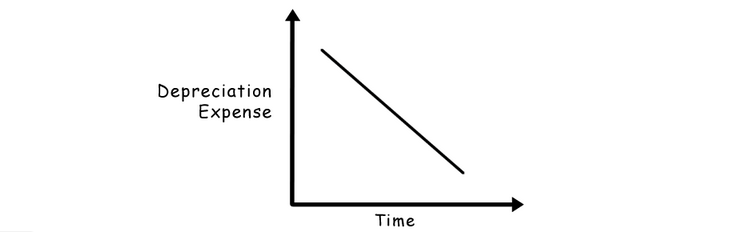The formula used is:

Depreciation = (Remaining Use life of an Asset/ Sum of Years Digit) * Total cost of an Asset

Here, the total cost of an asset is 1500; the remaining life of an asset = 5 years and the sum of years digit for years  1 + 2 + 3 + 4 + 5 = 15.

So, the total cost of an asset * remaining life of an asset/sum of years digit.

1st year:

1500 * 5/ 15 = 500;

2nd year:

1500 *4/ 15 = 400;

3rd year:

1500 * 3/ 15 = 300;

4th year:

1500 * 2/ 15 = 200

5th year:

1500 * 1/ 15 = 100.

Double Declining Balancing Method (DDB)

When using this method, assets depreciate twice as fast as the usual decreasing balance method. It also considers that depreciation charges are higher in the early years of an asset's life and lower in the later years.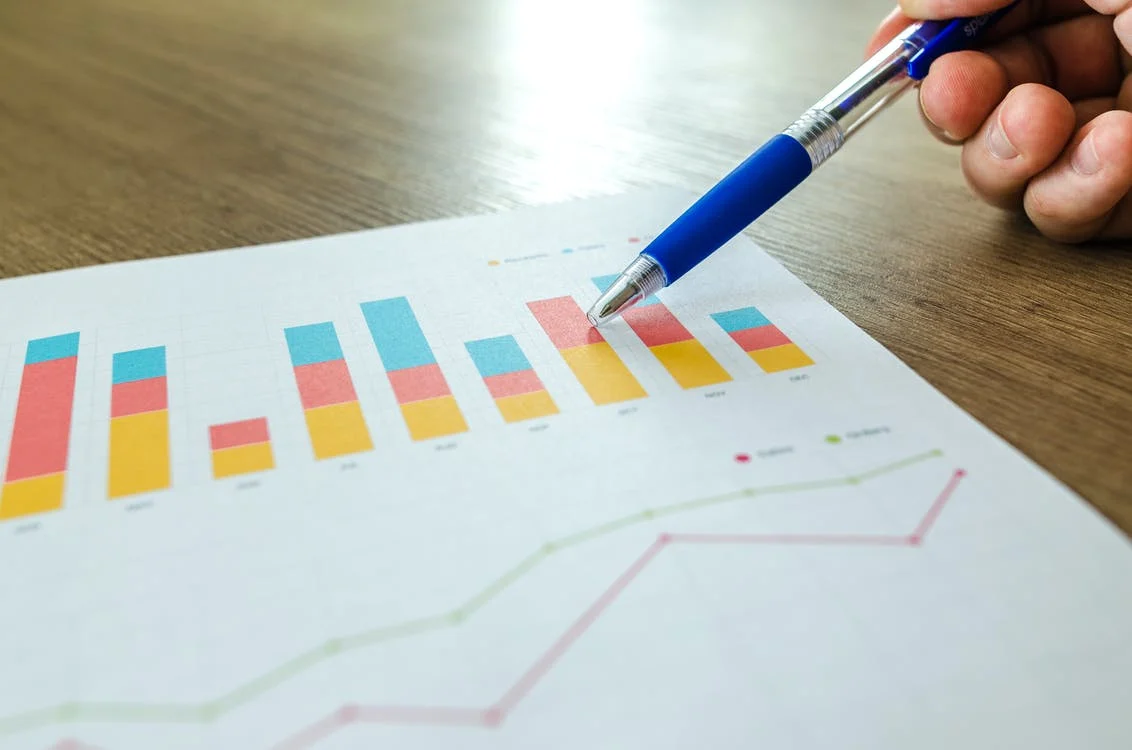The formula used is:

Depreciation = Depreciation Rate(%) * Book Value

Depreciation rate = 2 * (1/ Useful life of an asset)

= 2 * (1/5) = 0.4%

Applying the formula, here are the decomposition of depreciation and book value:

1st year:

Depreciation: 0.4 * 2000 = 800

Book value: 2000-800=1200;

2nd year:

Depreciation: 0.4 * 1200 = 480;

Book value: 1200-480=720;

3rd year:

Depreciation: 0.4 * 720 = 288;

Book value: 720-288=432;

4th year:

Depreciation: 0.4 * 432 = 173

Book value: 432-173=259;

5th year:

Depreciation: 0.4 * 173 = 69

Book value: 259-69=190.

Straight Line Depreciation (SLD)

Depreciation is calculated using a straight line technique in which the value of a fixed asset is diminished during its useful life.

The total cost of an asset is \$2,000, the residual value is \$500, and the estimated lifespan is five years.

Depreciation = (Cost of an asset - Residual Value) / (Estimated useful life of an asset)

= (2000 - 5005)/ 5 = 1500/ 5

=\$300

Here, in SLD, every year, the MacBook depreciates by \$300.

### Journal Entries For Sum Of The Years Digits Depreciation

The journal entry for depreciation is an adjusting entry, and it's the one to make before running an adjusted trial balance.

Make sure you've set up a contra asset account for your accumulated depreciation before making a journal entry. This account will track your accumulated depreciation expense entries to date.

From the previous example, the company debits the depreciation account and credits accumulated depreciation.

Journal Entry
YearJournal EntryDebitCredit
1Depreciation Expense A/c\$1,366,667-
-To Accumulated Depreciation A/c (Being the Syd for 1st year)-\$1,366,667
2Depreciation Expense A/c\$728,889-
-To Accumulated Depreciation A/c (Being the Syd for 2nd year)-\$728,889
3Depreciation Expense A/c\$400,889-
-To Accumulated Depreciation A/c (Being the Syd for 3rd year)-\$400,889
4Depreciation Expense A/c\$213,807-
-To Accumulated Depreciation A/c (Being the Syd for 4th year)-\$213,807
5Depreciation Expense A/c\$92,650-
-To Accumulated Depreciation A/c (Being the Syd for 5th year)-\$92,650

• This method assists in aligning the asset cost with its use every year over the asset's useful life. As the asset is most beneficial in its early years, this technique appears more realistic.

• This method balances depreciation expenses with other surplus expenses such as maintenance and repairs. In addition, it eliminates calculation errors made in the process.

• It serves as a tax shelter because the depreciation is larger in the first years, the net income reduces in the coming years, and the tax liability is lower.

• This is an accelerated method. The earnings may be disrupted or distorted if an accelerated approach is not used in situations where the asset would be most used in its early years. They may change since the initial depreciation expense will be lower; moreover, additional repairs and maintenance expenses will result in a decline in earnings.

• In the early years, it has a greater depreciation expense. As a result, profits in that period are reduced.

• Depreciation expenses are decreasing. The depreciation expense that declines is frequently countered by an increase in maintenance expense, smoothing out the revenue over time.

• It may have an indirect impact on cash flows.

• In comparison to the straight line, it may be more confusing and difficult to calculate.

### FAQ’s